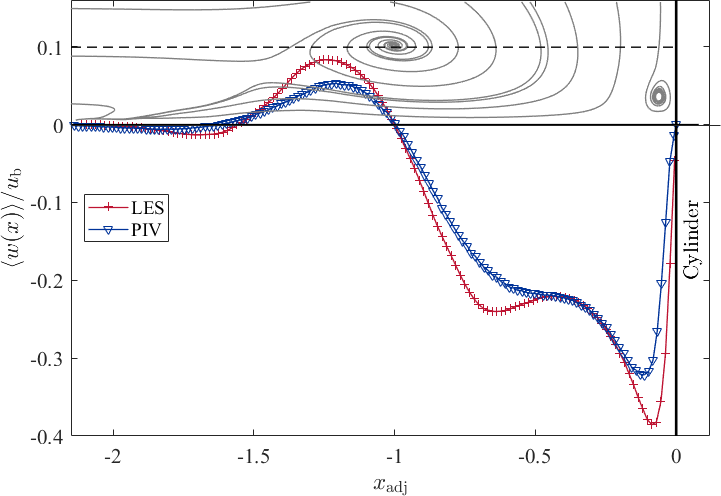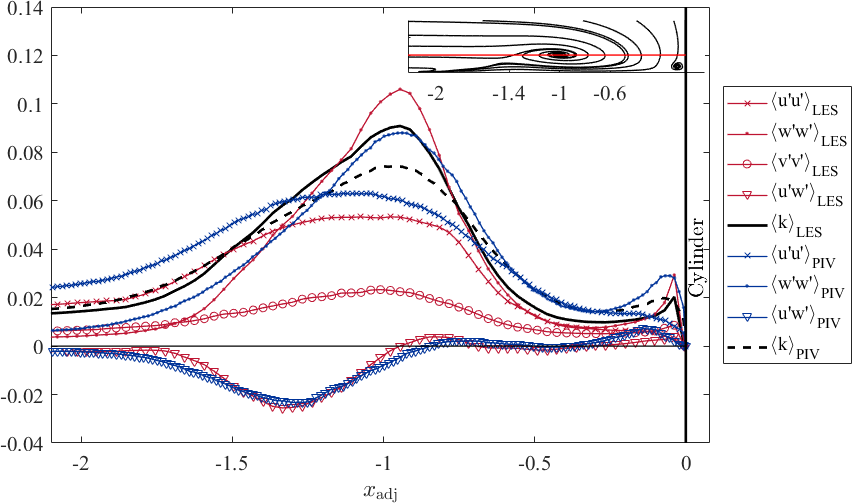# UFR 3-35 Evaluation

Jump to navigation Jump to search

# Evaluation

The evaluation of the numerical and experimental data sets in the symmetry plane upstream a wall-mounted cylinder refers to the time-averaged flow fields indicated by:

• the streamlines
• the location of the characteristic flow structures
• selected vertical and horizontal profiles of the velocity components $\langle u(z)\rangle$and $\langle w(x)\rangle$as well as the Reynolds sresses $\langle u'_{i}u'_{j}\rangle$and the trubulent kinetic energy $\langle k(z)\rangle$• the distribution of the turbulent kinetic energy and its buget terms such as production, transport, and dissipation
• horizontal profiles of the pressure coefficient $c_{\mathrm {p} }(x)$, and the friction coefficient $c_{\mathrm {f} }(x)$,

## Streamlines

The streamlines of the PIV and the LES data show high similarity and agree well with each other. The approaching turbulent boundary layer is redirected downwards at the flow facing edge of the cylinder caused by a vertical pressure gradient. This downflow reaches the bottom plate of the flume at the stagnation point S3. Here, it becomes redirected (i) in the out-of-plane direction bending around the cylinder; (ii) towards the cylinder rolling up and forming the corner vortex V3; and (iii) in the upstream direction accelerating and fomring a wall-parallel jet. Due to the strong acceleration of the jet, a high vertical velocity gradient is exerted onto the bottom plate, which induces high wall shear stress. Parts of the approaching boundary layer is dragged downwards forming the horseshoe vortex V1. Upstream of thsi vortex system, the approaching flow is blocked and recirculates, and as a consequence a saddle point S1 with zero velocity magnitude appears.

## Location of the characteristic flow structures

The position of the characteristic flow structures highlited in the streamline plots is listed in the following table

Position of flow structures
PIV LES
$x/D$$z/D$$x/D$$z/D$S1 $-0.788$$0.03$$-0.843$$0.037$S2 $-0.918$$0$$-1.1$$0$S3 $-0.533$$0$$-0.534$$0$S4 $-0.507$$0.036$$-0.50$$0.04$V1 $-0.697$$0.051$$-0.735$$0.06$V3 $-0.513$$0.017$$-0.513$$0.02$## Horizontal and vertical profiles of the velocity components and Reynolds stresses

The vertical profiles are extracted at the following positions with respect to the time-averaged position of the horseshoe vortex, and can be found in the following plot.

Since the flow structure of the LES and the PIV is slightly different, we use an adjusted $x-$coordinate, in order to compare the data at the same position in the flow. The coordinate is defined as follows:

$x_{\mathrm {adj} }={\frac {x-x_{\mathrm {Cyl} }}{x_{\mathrm {Cyl} }-x_{\mathrm {V1} }}}$,

with $x_{\mathrm {Cyl} }=-0.5D$, such that $x_{\mathrm {adj} }=-1.0$represents the time-averaged location of the horseshoe vortex centre $x_{\mathrm {V1} }$.

The vertical velcoity profiles $u(z)$are presented at the seleted locations. When the downflow is deflected at the bottom plate at $S3$, the wall-parallel jet starts to develop, and consequently, the flow accelerates. At $x_{\mathrm {adj} }=-0.25$, a near-wall velocity peak appears, which becomes more pronounced in the upstream direction (see $x_{\mathrm {adj} }=-0.5$). In this region, the wall shear stress reaches on one hand its maximum value and on the other hand reveals a plateau-like shape. This means that the largest values of the gradient ${\frac {\partial \langle u\rangle }{\partial z}}$appear. Underneath the horseshoe vortex, the flow decelerates and the wall-peak of the velocity lifts from the bottom plate and becomes less distinct. Further upstream, the near-wall peak of the streamwise velocity disappears and therefore, the wall-parallel jet fades out. Due to the evaluation of the PIV images by an interrogation window based cross correlation, the strong gradient ${\frac {\partial \langle u\rangle }{\partial z}}$at the wall cannot be fully resolved, and therefore, the near-wall peak of the streamwise velocity is damped in the experimental data.

The vertical profiles of the Reynolds stresses and the resulting turbulent kinetic eneryg is presented only at $x_{\mathrm {adj} }=-1.5$, $x_{\mathrm {adj} }=-1.0$, and $x_{\mathrm {adj} }=-0.5$. The accelerating jet is again indicated by a near wall peak of the $\langle u'u'\rangle$stress, while the horseshoe vortex leaves its footprint in the stresses $\langle u'u'\rangle$and $\langle w'w'\rangle$as (local) peak at $z_{\mathrm {V1} }/D$. The shear stress distribution $\langle u'w'\rangle$inside the wall-paralle jet is negative (in average) according to the flow direction (in average). The experimental and numerical data agree with each other both in amplitude as well as in shape. Again, the quality of the PIV data near the wall is underminded by the strong gradients being evaluated using interrogation windows.Horizontal profile of $\langle w(x)/\rangle /u_{\mathrm {b} }$at the height of the horseshoe vortex centre for LES and PIVHorizontal profile of $\langle u'u'\rangle /u_{\mathrm {b} ^{2}}$at the height of the horseshoe vortex centre for LES and PIV

## Horizontal profiles of the pressure coefficient $c_{\mathrm {p} }(x)$Contributed by: Ulrich Jenssen, Wolfgang Schanderl, Michael Manhart — Technical University Munich

© copyright ERCOFTAC 2019